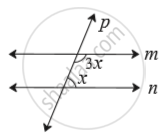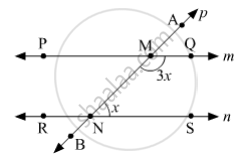SSC (English Medium) Class 8Maharashtra State Board
Share

# Choose the Correct Alternative. in the Adjoining Figure, If Line M Line N and Line P is a Transversal Then Find X. (A) 135° (B) 90° (C) 45° (D) 40° - SSC (English Medium) Class 8 - Mathematics

#### Question

Choose the correct alternative.

In the adjoining figure, if line m || line n and line p is a transversal then find x.• 135°

• 90°

• 45°

• 40°

#### SolutionLet us mark the points P and Q on m; R and S on n; A and B on p.
Suppose PQ and AB intersect at M; RS and AB intersect at N.
Since, m||n and p is a transversal, then
m∠QMN + m∠SNM = 180°    (Interior angles on the same side of transversal are supplementary)
Substituing the values in the above equation, we get
3x + x = 180°
⇒ 4x = 180°
⇒ x = (180°)/4
∴ x = 45°
So, the correct answer is option (C).

Is there an error in this question or solution?

#### APPEARS IN

Balbharati Solution for Balbharati Class 8 Mathematics (2019 to Current)
Chapter 2: Parallel lines and transversals
Practice Set 2.2 | Q: 1.1 | Page no. 11
Solution Choose the Correct Alternative. in the Adjoining Figure, If Line M Line N and Line P is a Transversal Then Find X. (A) 135° (B) 90° (C) 45° (D) 40° Concept: Transversal.
S